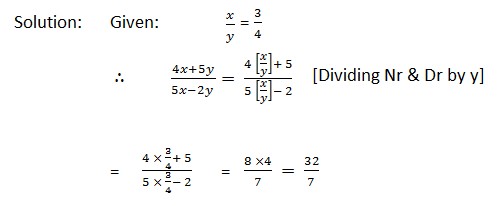Gurpurab Offer - Use Code GURPURAB2023

# Ratio and Proportions questions for SBI Clerk

Ratio and Proportions questions for IBPS PO :-Must read Ratio and Proportions shortcuts here.

#### Ques 1.

If a: b = 5 : 9 and b : c = 4 : 7, find a : b : c and a : c.#### Ques 2.

If x : y = 3 : 4, find (4x + 5y) : (5x – 2y).#### Ques 3.

Divide Rs. 672 in the ratio 5 : 3.
Solution: Sum of the terms of the ratio = (5 + 3) = 8

First part = Rs. (672 × 5/8) = Rs. 420

Second part = Rs. (672 × 3/8) = Rs. 252

#### Ques 4.

Divide Rs. 1162 among A, B, and C in the ratio 35 : 28 : 20.
Solution: Sum of the terms of the ratio = (35 + 28 + 20) = 83.

A’s share = Rs. (1162 × 35/83) = Rs. 490.

B’s share = Rs. (1162 × 28/83) = Rs. 392.

C’s share = [1162 – (490 + 392)] = Rs. 280.

#### Ques 5.

A bag contains 50 paise, 25 paise and 10 paise coins in the ratio 5 : 9 : 4, amounting to Rs. 206. Find the number of coins of each type.#### Ques 6.

Which one of 2 : 3, 4 : 5, 7 : 9 and 11 : 13 is the largest ratio?
Solution: 2 : 3 = 2/3=0.666;   4 : 5 = 4/5 = 0.8;

7 : 9 = 7/9 = 0.777; 11 : 13 = 11/13 = 0.846

Clearly,  0.846 > 0.8 > 0.777 > 0.666.

Hence,  11 : 13 is the largest ratio.

#### Ques 7.

A mixture contains alcohol and water in the ratio 4 : 3. If 5 litres of water is added to the mixture, the ratio becomes 4 : 5. Find the quantity of alcohol in the given mixture.#### Ques 8.

Suppose x varies as the sum of two quantities of which one varies directly as x and the other inversely as x. If y = 6 when x = 4 and y = 3 1/3, when x = 3. Find the relation between x and y.